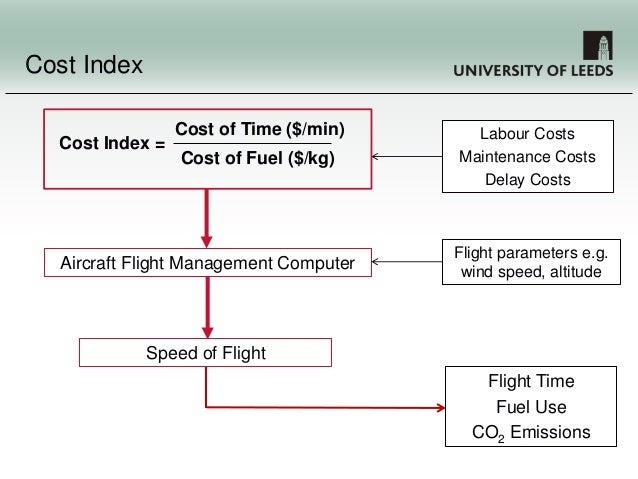## Different Cost indexes from alot of airlines

Angel Wings Pilots Discussion About All Software Needed For Fliying.

Moderators: MAURO BOCCELLI, Luca Nicolini

###Different Cost indexes from alot of airlinesThe cost index is a number used in the Flight Management System (FMS) to optimize the aircraft's speed. It gives the ratio between the unit cost of time and the unit cost of fuel.
With this number, and knowledge about the aircraft's performance, it is possible to calculate the optimal speed for the aircraft, which results in the lowest total cost.
Speeds slower than the optimal speed will result in less fuel burn, but also in more flying time. The cost of the extra flying time outweighs the fuel savings at speeds below the optimum speed.
Speeds faster than the optimal speed will result in more fuel burn, but also in less flying time. The saving of less flight time do not outweigh the fuel burn at speeds above the optimum speed.
A low cost index means that the cost of time is low or that fuel is expensive. It will result in a low speed. High cost index means high cost of time (e.g. passengers about to miss their flight connection) or low fuel price (rare these days).
At the minimum cost index (0) only fuel counts. This will result in the aircraft flying at Maximum Range Cruise. At the maximum cost index only time counts. This will result in the aircraft flying at Maximum Cruise Speed (Vmo / Mmo with a buffer)

Airlines generally have a standard cost index they use for planning and adjust them on a flight by flight basis.

SAS:

*Boeing 737 = CI 6
*CRJ9 = CI 9
*A319/320/321 = CI 6
*A330/340 = CI 50

Norwegian Air Shuttle:

*B737-800 = CI 15/80(DELAY ONLY)
*B737-300 = CI 30
*B787-800 = CI 36

British Airways BA/BAW:

*A318 = CI 30
*A319 = CI 15
*A320 = CI 15
*A321 - CI 20
*B744 = CI 50
*B763 = CI 40
*B772 = CI 100
*B773 = CI 100
*B787 = CI 25
*A380 = CI 120

TAM Linheas Areàs JJ/TAM:

*A319 = CI 19
*A320 = CI 26 A321 = CI 25
*B763 = CI 34
*B77W = CI 38 A332 = CI 26

EasyJet U2/EZY/EZS:

*A319/A320/A320SL = CI 12

Wizzair W6/WZZ:

*A320/A320SL = CI 15

Aegean Airlines A3/AEE:

*A320 = CI 20
*A321 = CI 25

Royal Air Maroc AT/RAM:

*B737 = CI 34
*B738 = CI 24 / CI 50 (to/from LHR,ORY,AMS)
*B763 = CI 39
*B788 = CI 42

Nouvelair Tunise BJ/LBT:

*A320 = CI 38

Tunisair TU/TAR:

*A320 = CI 17
*B736 = CI 13

Luxair LG/LGL:

*B737 = CI 10 B738 = CI 8

Icelandair FI/ICE:

*B757-300 = CI 18 B757-200 = CI 16

Thomson Airways BY/TOM:

*Boeing 787 = CI 21 Boeing 737 = CI 13 Boeing 757 = CI 23 Boeing 767 = CI 25

Air Berlin AB/BER:

*Airbus A320/NLY = CI 8 (used on OE-xxx registered planes) Airbus A321/NLY = CI 7 (used on OE-xxx registered planes) Airbus A319/BHP = CI 3 (used on HB-xxx registered planes) Airbus A319/BER = CI 6 (Used on D-xxxx registered planes) Airbus A320/BER = CI 8 (Used on D-xxxx registered planes) Airbus A321/BER = CI 8 (Used on D-xxxx registered planes) Boeing 737-700 = CI 8 Boeing 737-800 = CI 8 Airbus A330-200 = CI 80

Emirates EK/UAE:

*Airbus A330-200= CI 26 Airbus A340-300= CI 24 Airbus A340-500= CI 28 Airbus A380-800= CI 50 B777-300ER = CI 36 B777-300ERD = CI 37 (ICAO Type : B773D, reg: A6EMN, EMM, EMO, EMP, EMR, EMS, EMT, EMU, EMV, EMX and EMW) B777-200LR/F = CI 35 B777-200ER = CI 36 B777-200 = CI 34 (Used on short haul flights only, ICAO Type B772 reg: A6EMI, A6EMH, A6EML,A6EMK and A6EMJ)

Qatar Airways QR/QTR:

*Airbus A319CJ = CI 9 (Outbound from DOH) / CI 8 (Inbound to DOH) Airbus A320 = CI 10 Airbus A321 = CI 11 Airbus A330-200 = CI 15 Airbus A330-300 = CI 15 Airbus A330-200F = CI 15 Airbus A340-600 = CI 21 Airbus A350-900 = CI 30
*Airbus A380-800 = CI160
*Boeing 777-200LR = CI 120 (Outbound from DOH) / CI 110 (Inbound to DOH) Boeing 777-200LRF = CI 120 Boeing 777-300ER = CI 120 Boeiing 787-800 = CI 110 (Outbound from DOH) / CI 25 (Inbound to DOH)

Egyptair MS/MSR:

*B737-500 = 18 B737-800 = CI 22 A320 = CI 23 A321 = CI 25 A330-200/300 = CI 35 B772 = CI 37 B77W = CI 44

Thomas Cook Airlines Belgium HQ/TCW:

*A320 = CI 10

Thomas Cook Airlines UK MT/TCX:

*A321 = CI 13 A332 = CI 27 B752 = CI 28 B753 = CI 29 B763 = CI 27

Thomas Cook Airlines Scandinavia DK/VKG:

*A321 = CI 21 A332 = CI 40 A333 = CI 39

Condor DE/CFG:

*A320 = CI 26 A321 = CI 33 B753 = CI 30 B763 = CI 30

Novair 1I/NVR:

*A321 = CI 4

Etihad Airlines EY/ETD:

*A319 = CI 18 A320 = CI 16 A320SL = CI 18 A321SL = CI 15 A332 = CI 23 A332F = CI 25 A333 = CI 26 A345 = CI 32 A346 = CI 32 B772LR = CI 41 B772LRF = CI 44 B77W (Only reg A6-JAx series) = CI 43 B77W (Only reg A6-ETx series) = CI 45 B789 = CI 25
*A388 = CI 50

ALL OF THE ABOVE IS TAKEN FROM REAL OFPS!

SO IT IS 100% ACCURATEGiacomo Adami
Site AdminPosts: 86
Joined: 21 May 2012, 15:05
Location: Italy

Return to Pilots Software & Resources (ENG)